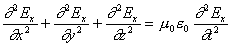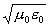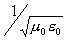Teaching Physics with the Physics Suite Edward F. Redish

Properties of EM waves

The wave equation for one component of the electric field in an electromagnetic wave isBelow are listed two situations and a number of statements about the field that solves this equation. For each of the situations A and B indicate which of the answers a-f must be true.

Situations:

(A) The electromagnetic wave considered is approximately a plane wave traveling in the +z direction.
(B) The electromagnetic wave considered is approximately a single pulse (a rise of the magnitude of E from zero followed by a fall back to zero) moving in the -y direction.

Statements:

1. The wave is polarized in the x direction.
2. The electric field vector at any instant of time is (nearly) independent of z.
3. The solution does not appear to change if we move in the direction of travel at a speed4. The solution does not appear to change if we move in the direction of travel at a speed5. The graph of (at least) one component of the electric field as observed at a fixed point in space will oscillate like a sine or cosine function as the wave passes that point.
6. The graph of (at least) one component of the electric field as observed at many points in space at a fixed time will oscillate like a sine or cosine function.

Not finding what you wanted? Check the Site Map for more information.